# Data Types and Variables Numeral Types Text Types

• Slides: 57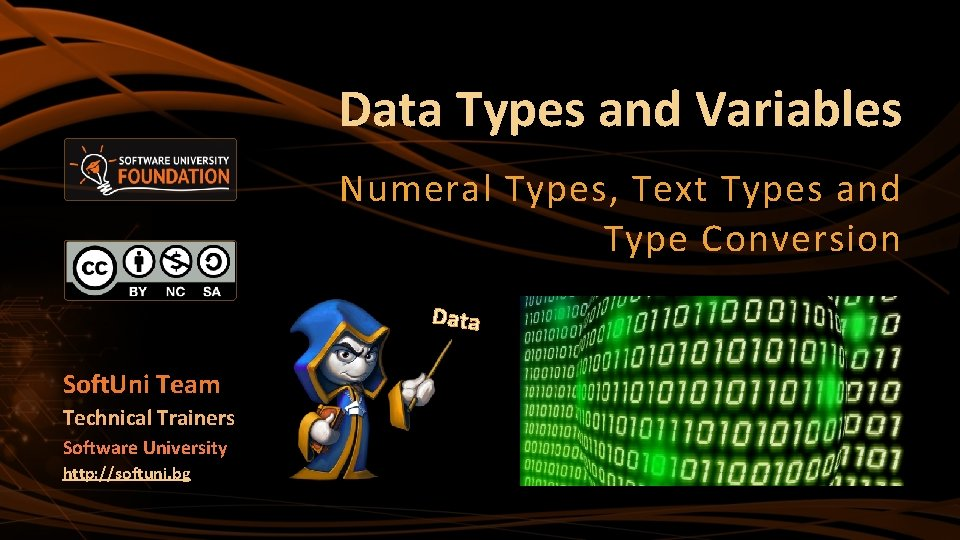Data Types and Variables Numeral Types, Text Types and Type Conversion Data Soft. Uni Team Technical Trainers Software University http: //softuni. bg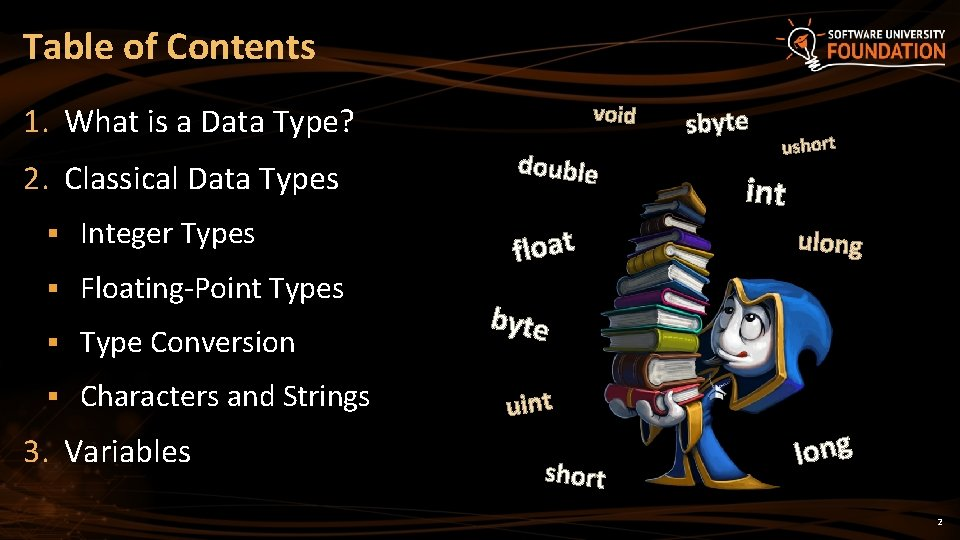Table of Contents void 1. What is a Data Type? 2. Classical Data Types § Integer Types § Floating-Point Types § Type Conversion § Characters and Strings 3. Variables double float sbyte ushort int ulong byte uint short long 2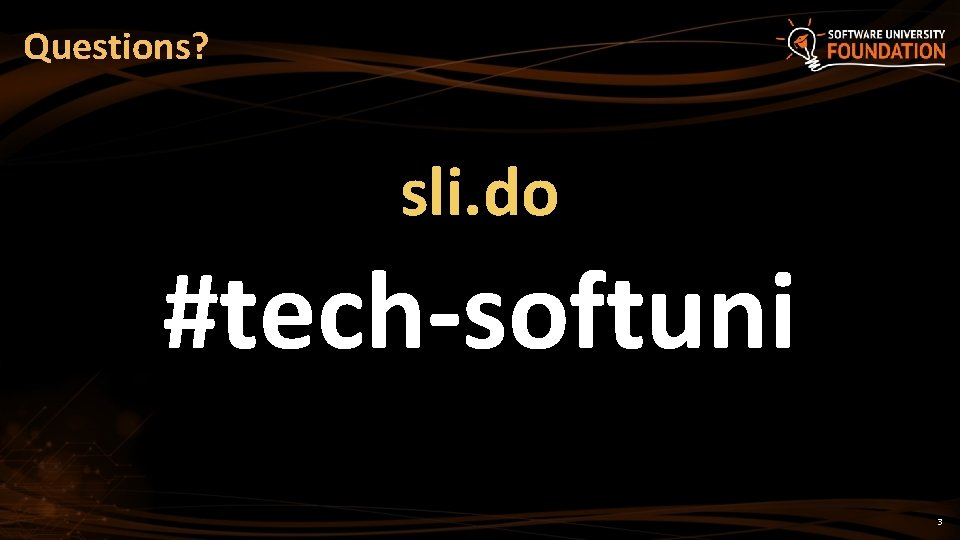Questions? sli. do #tech-softuni 3Data Types, Variables and Type ConversionsHow Computing Works? § Computers are machines that process data § Instructions and data are stored in the computer memory 10110 5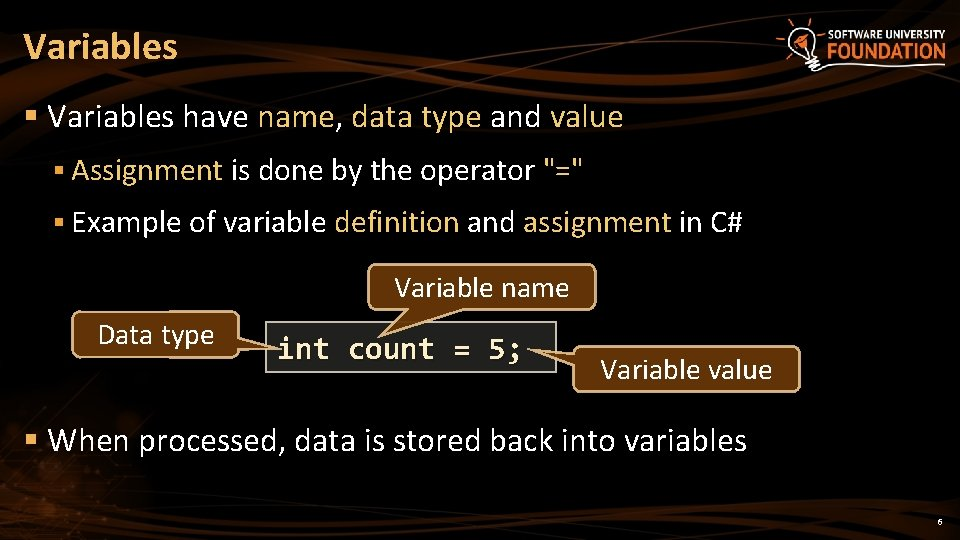Variables § Variables have name, data type and value § Assignment is done by the operator "=" § Example of variable definition and assignment in C# Variable name Data type int count = 5; Variable value § When processed, data is stored back into variables 6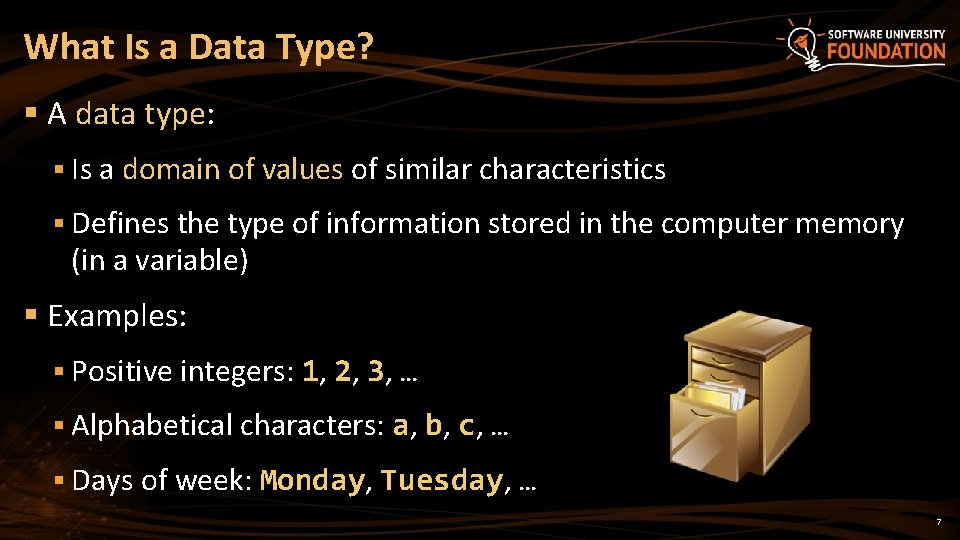What Is a Data Type? § A data type: § Is a domain of values of similar characteristics § Defines the type of information stored in the computer memory (in a variable) § Examples: § Positive integers: 1, 2, 3, … § Alphabetical characters: a, b, c, … § Days of week: Monday, Tuesday, … 7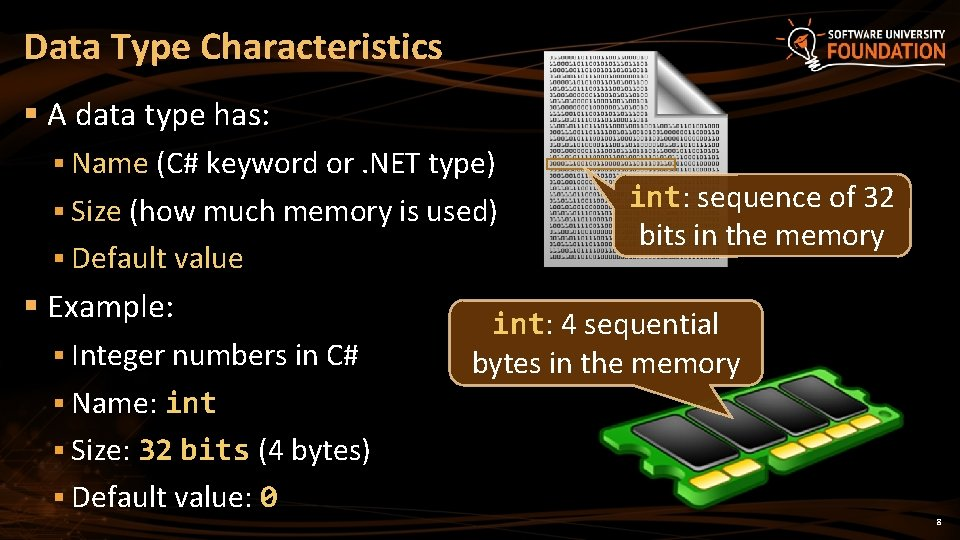Data Type Characteristics § A data type has: § Name (C# keyword or. NET type) § Size (how much memory is used) § Default value § Example: § Integer numbers in C# int: sequence of 32 bits in the memory int: 4 sequential bytes in the memory § Name: int § Size: 32 bits (4 bytes) § Default value: 0 8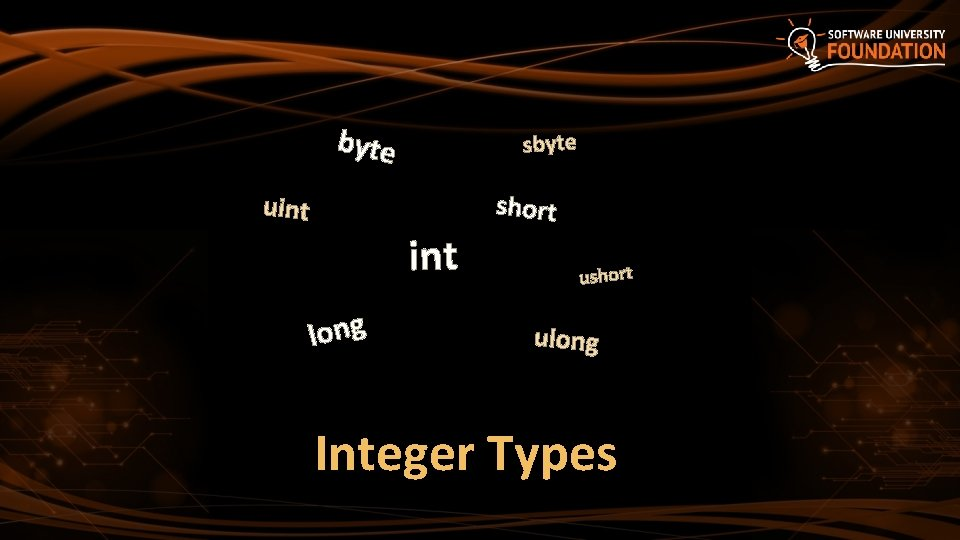byte uint sbyte int long short ulong Integer Types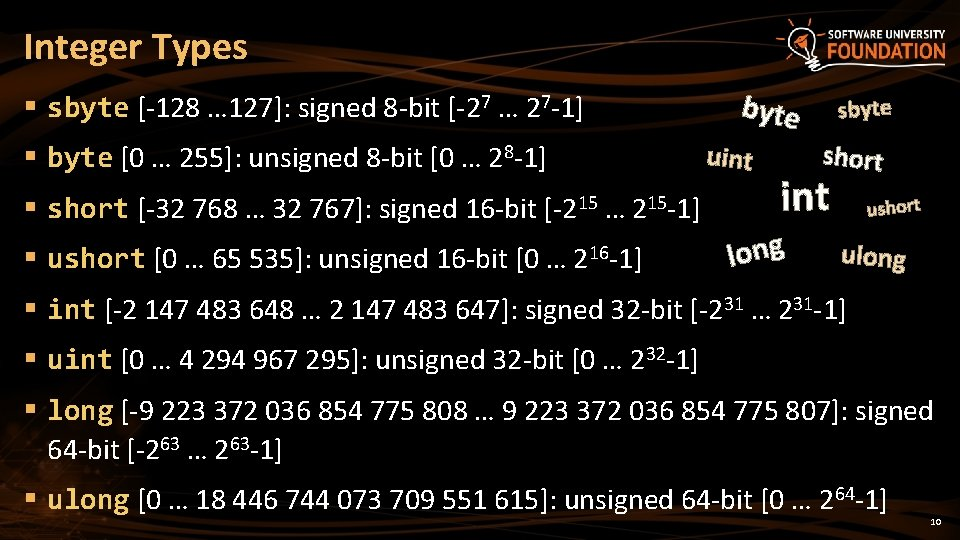Integer Types § sbyte [-128 … 127]: signed 8 -bit [-27 … 27 -1] § byte [0 … 255]: unsigned 8 -bit [0 … 28 -1] § short [-32 768 … 32 767]: signed 16 -bit [-215 … 215 -1] § ushort [0 … 65 535]: unsigned 16 -bit [0 … 216 -1] byte uint sbyte short int long ushort ulong § int [-2 147 483 648 … 2 147 483 647]: signed 32 -bit [-231 … 231 -1] § uint [0 … 4 294 967 295]: unsigned 32 -bit [0 … 232 -1] § long [-9 223 372 036 854 775 808 … 9 223 372 036 854 775 807]: signed 64 -bit [-263 … 263 -1] § ulong [0 … 18 446 744 073 709 551 615]: unsigned 64 -bit [0 … 2 64 -1] 10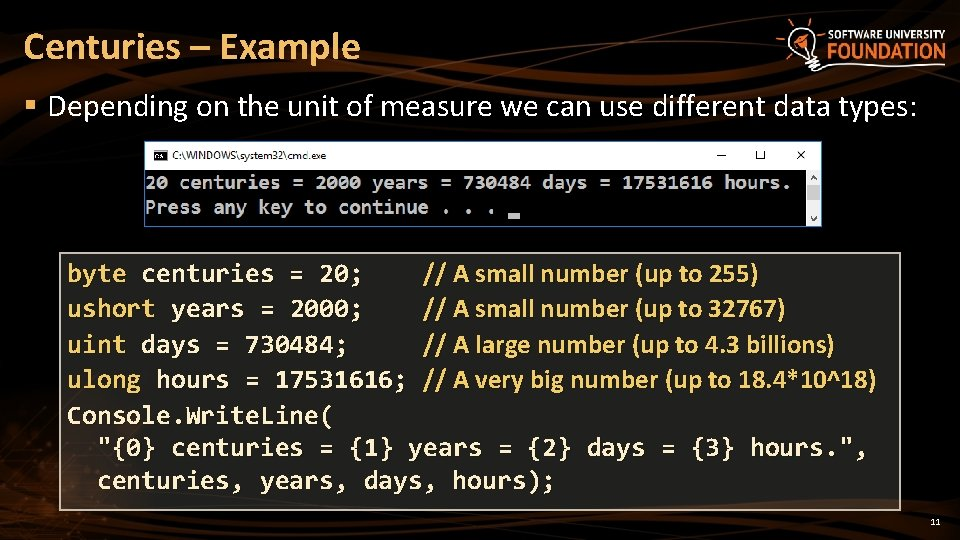Centuries – Example § Depending on the unit of measure we can use different data types: byte centuries = 20; // A small number (up to 255) ushort years = 2000; // A small number (up to 32767) uint days = 730484; // A large number (up to 4. 3 billions) ulong hours = 17531616; // A very big number (up to 18. 4*10^18) Console. Write. Line( "{0} centuries = {1} years = {2} days = {3} hours. ", centuries, years, days, hours); 11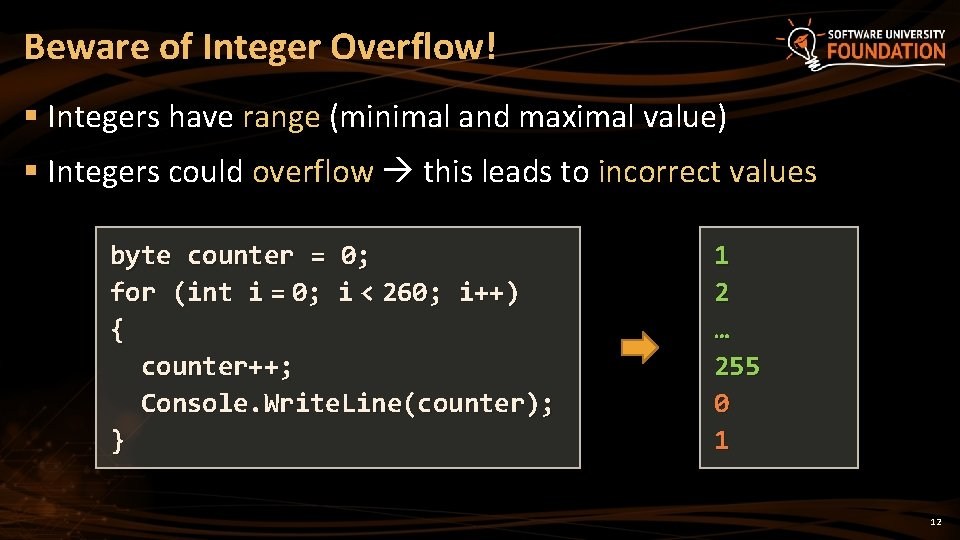Beware of Integer Overflow! § Integers have range (minimal and maximal value) § Integers could overflow this leads to incorrect values byte counter = 0; for (int i = 0; i < 260; i++) { counter++; Console. Write. Line(counter); } 1 2 … 255 0 1 12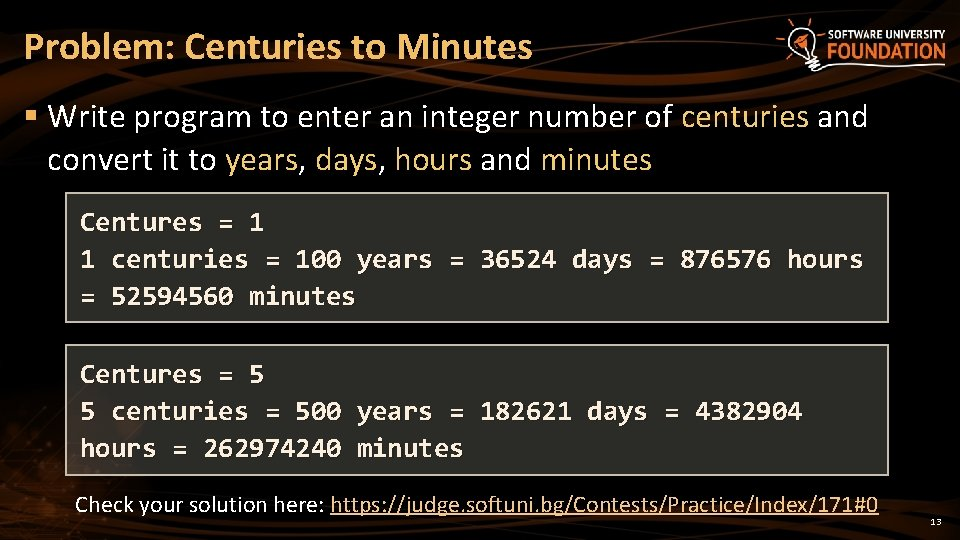Problem: Centuries to Minutes § Write program to enter an integer number of centuries and convert it to years, days, hours and minutes Centures = 1 1 centuries = 100 years = 36524 days = 876576 hours = 52594560 minutes Centures = 5 5 centuries = 500 years = 182621 days = 4382904 hours = 262974240 minutes Check your solution here: https: //judge. softuni. bg/Contests/Practice/Index/171#0 13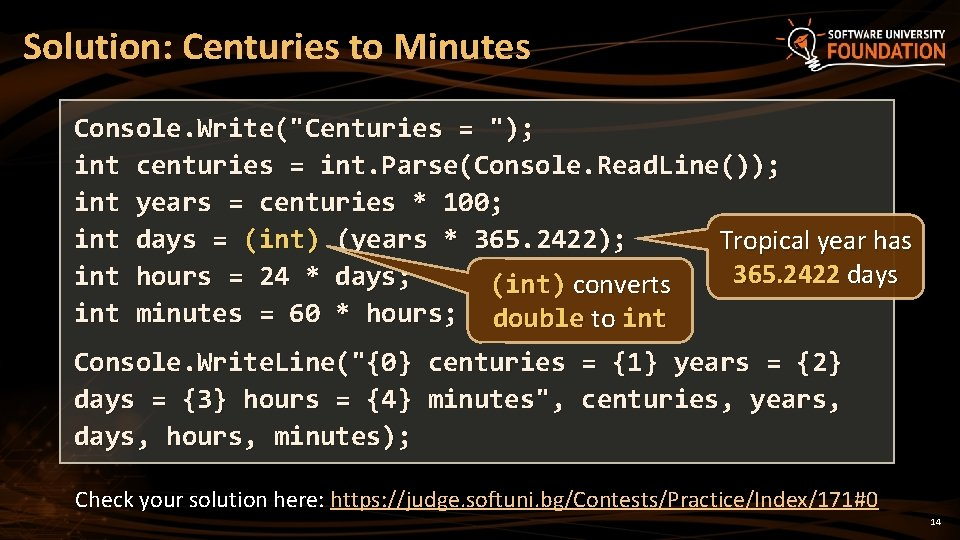Solution: Centuries to Minutes Console. Write("Centuries = "); int centuries = int. Parse(Console. Read. Line()); int years = centuries * 100; int days = (int) (years * 365. 2422); Tropical year has 365. 2422 days int hours = 24 * days; (int) converts int minutes = 60 * hours; double to int Console. Write. Line("{0} centuries = {1} years = {2} days = {3} hours = {4} minutes", centuries, years, days, hours, minutes); Check your solution here: https: //judge. softuni. bg/Contests/Practice/Index/171#0 14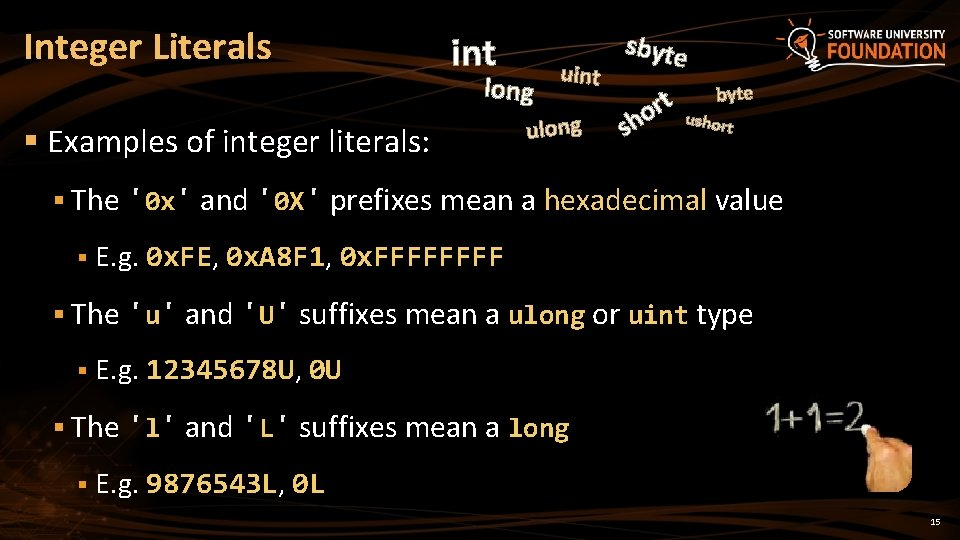Integer Literals int long § Examples of integer literals: uint ulong sbyte rt o sh byte ushor t § The '0 x' and '0 X' prefixes mean a hexadecimal value § E. g. 0 x. FE, 0 x. A 8 F 1, 0 x. FFFF § The 'u' and 'U' suffixes mean a ulong or uint type § E. g. 12345678 U, 0 U § The 'l' and 'L' suffixes mean a long § E. g. 9876543 L, 0 L 15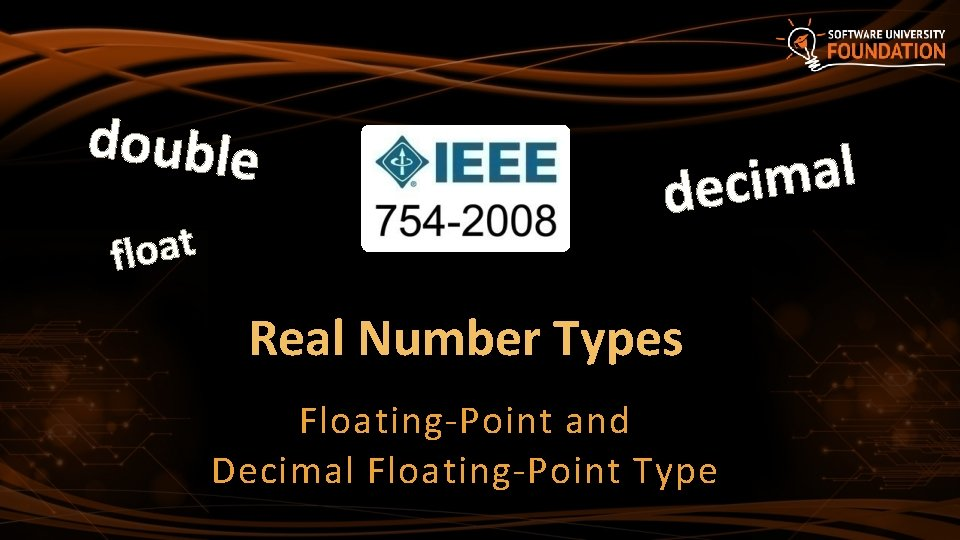double float l a m i dec Real Number Types Floating-Point and Decimal Floating-Point TypeWhat are Floating-Point Types? § Floating-point types: e l b u o d § Represent real numbers, e. g. 1. 25, -0. 38 float § Have range and precision depending on the memory used § Sometimes behave abnormally in the calculations § May hold very small and very big values like 0. 00000001 and 100000000000000000. 0 17Floating-Point Numbers § Floating-point types are: § float (± 1. 5 × 10− 45 to ± 3. 4 × 1038) § 32 -bits, precision of 7 digits § double (± 5. 0 × 10− 324 to ± 1. 7 × 10308) § 64 -bits, precision of 15 -16 digits double t a o l f § The default value of floating-point types: § Is 0. 0 F for the float type § Is 0. 0 D for the double type 18PI Precision – Example § Difference in precision when using float and double: float. PI = 3. 141592653589793238 f; double. PI = 3. 141592653589793238; Console. Write. Line("Float PI is: {0}", float. PI); Console. Write. Line("Double PI is: {0}", double. PI); § NOTE: The "f" suffix in the first statement! § Real numbers are by default interpreted as double! § One should explicitly convert them to float 19Rounding Floating-Point Numbers § Math. Round(3. 45) – round to integer number (mathematically) § Math. Round(2. 3455, 3) – round with precision § Math. Ceiling() – round up to the nearest integer § Math. Floor() – round down to the nearest integer double a = 2. 3455; Console. Write. Line(Math. Round(a)); Console. Write. Line(Math. Round(a, 3)); Console. Write. Line(Math. Ceiling(a)); Console. Write. Line(Math. Floor(a)); // // Banker's rounding: 2. 5 3 3. 5 4 3. 45 3 result: 2 2. 346 3 2 20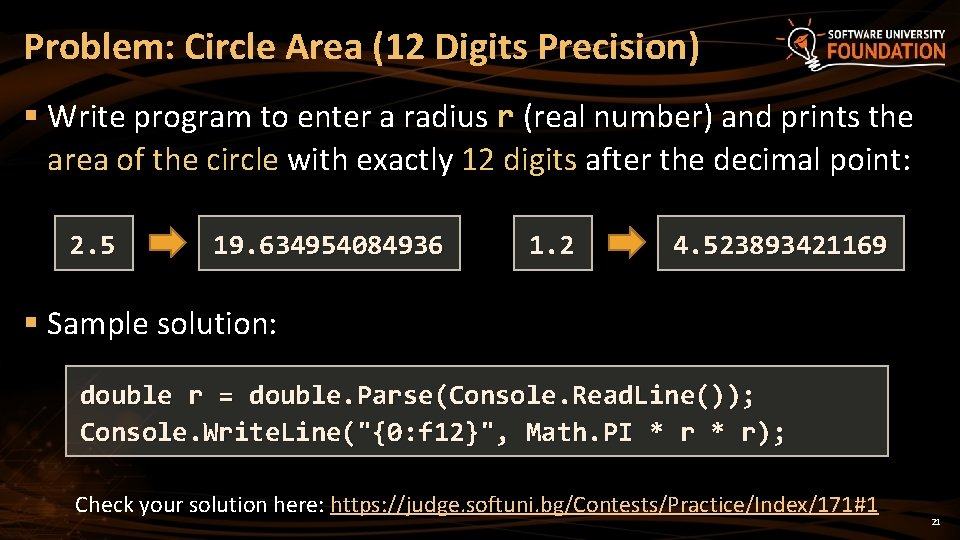Problem: Circle Area (12 Digits Precision) § Write program to enter a radius r (real number) and prints the area of the circle with exactly 12 digits after the decimal point: 2. 5 19. 634954084936 1. 2 4. 523893421169 § Sample solution: double r = double. Parse(Console. Read. Line()); Console. Write. Line("{0: f 12}", Math. PI * r); Check your solution here: https: //judge. softuni. bg/Contests/Practice/Index/171#1 21Scientific Notation § Floating-point numbers can use scientific notation, e. g. § 1 e+34, 1 E 34, 20 e-3, 1 e-12, -6. 02 e 28 double d = 100000000000000000. 0; Console. Write. Line(d); // 1 E+34 double d 2 = 20 e-3; Console. Write. Line(d 2); // 0. 02 double d 3 = double. Max. Value; Console. Write. Line(d 3); // 1. 79769313486232 E+308 22Floating-Point Division § Integral division and floating-point division are different: Console. Write. Line(10 / 4); Console. Write. Line(10 / 4. 0); Console. Write. Line(10 / 0. 0); Console. Write. Line(-10 / 0. 0); Console. Write. Line(8 % 2. 5); // // // 2 (integral division) 2. 5 (real division) Infinity -Infinity Na. N (not a number) 0. 5 (3 * 2. 5 + 0. 5 = 8) int d = 0; // Integral division works differently Console. Write. Line(10 / d); // Divide. By. Zero. Exception 23Floating-Point Calculations – Abnormalities § Sometimes floating-point numbers work incorrectly! Console. Write. Line(10000000. 0 + 0. 3); // Result: 10000000 (loss of precision) double a = 1. 0 f, b = 0. 33 f, sum = 1. 33; Console. Write. Line("a+b={0} sum={1} equal={2}", a+b, sum, (a+b == sum)); // a+b=1. 33000001311302 sum=1. 33 equal=False double one = 0; for (int i = 0; i < 10000; i++) one += 0. 0001; Console. Write. Line(one); // 0. 999999906 24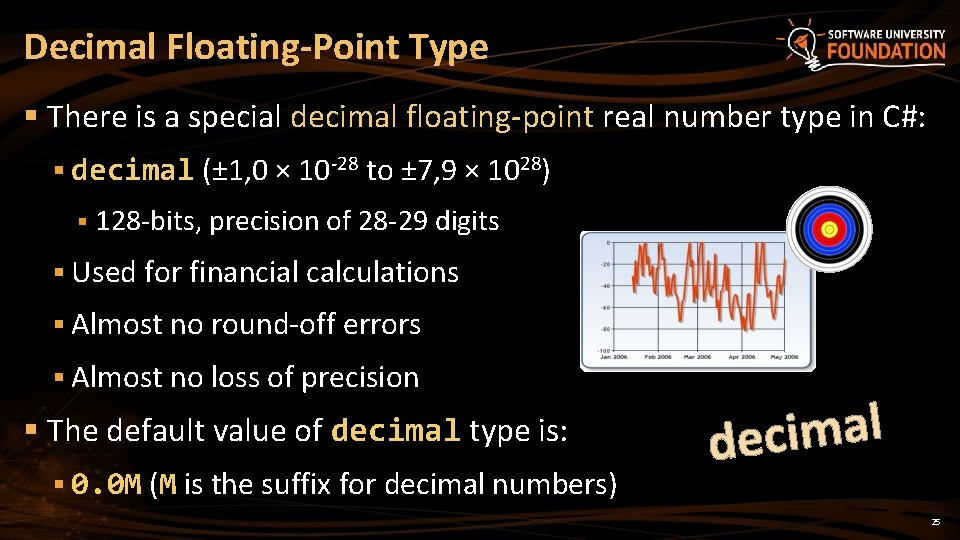Decimal Floating-Point Type § There is a special decimal floating-point real number type in C#: § decimal (± 1, 0 × 10 -28 to ± 7, 9 × 1028) § 128 -bits, precision of 28 -29 digits § Used for financial calculations § Almost no round-off errors § Almost no loss of precision § The default value of decimal type is: § 0. 0 M (M is the suffix for decimal numbers) l a m i dec 25Problem: Exact Sum of Real Numbers § Write program to enter n numbers and print their exact sum: 2 1000000000 5 10000000005 2 0. 000003 333333333333. 3000003 Check your solution here: https: //judge. softuni. bg/Contests/Practice/Index/171#2 26Solution: Exact Sum of Real Numbers § This code works but makes mistakes sometimes: int n = int. Parse(Console. Read. Line()); double sum = 0; for (int i = 0; i < n; i++) sum += double. Parse(Console. Read. Line()); Console. Write. Line(sum); § Change double with decimal and check the differences Check your solution here: https: //judge. softuni. bg/Contests/Practice/Index/171#2 27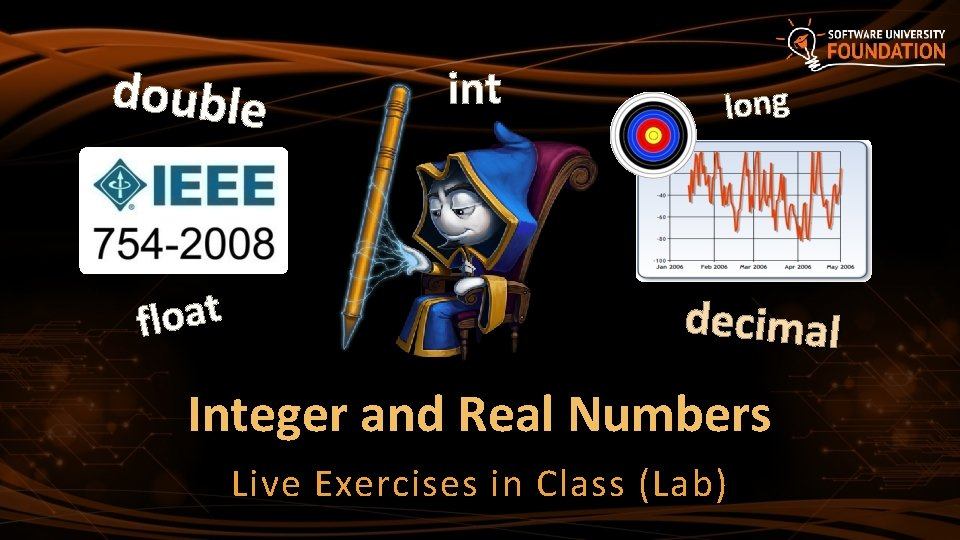double t a o l f int long decimal Integer and Real Numbers Live Exercises in Class (Lab)Type ConversionType Conversion § Variables hold values of certain type § Type can be changed (converted) to another type § Implicit type conversion (lossless): variable of bigger type (e. g. double) takes smaller value (e. g. float) float height. In. Meters = 1. 74 f; double max. Height = height. In. Meters; // Implicit conversion § Explicit type conversion (lossy) – when precision can be lost: double size = 3. 14; int. Size = (int) size; // Explicit conversion 3 30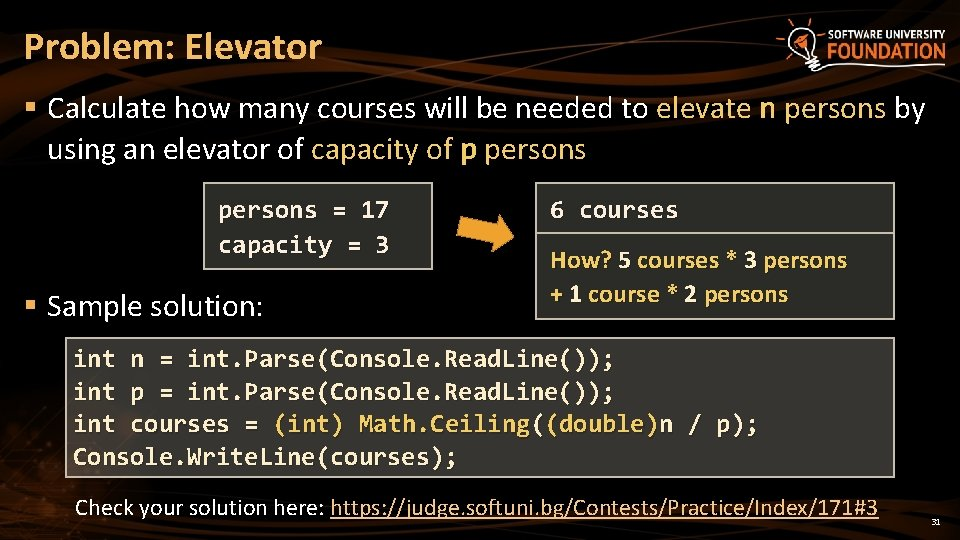Problem: Elevator § Calculate how many courses will be needed to elevate n persons by using an elevator of capacity of p persons = 17 capacity = 3 § Sample solution: 6 courses How? 5 courses * 3 persons + 1 course * 2 persons int n = int. Parse(Console. Read. Line()); int p = int. Parse(Console. Read. Line()); int courses = (int) Math. Ceiling((double)n / p); Console. Write. Line(courses); Check your solution here: https: //judge. softuni. bg/Contests/Practice/Index/171#3 31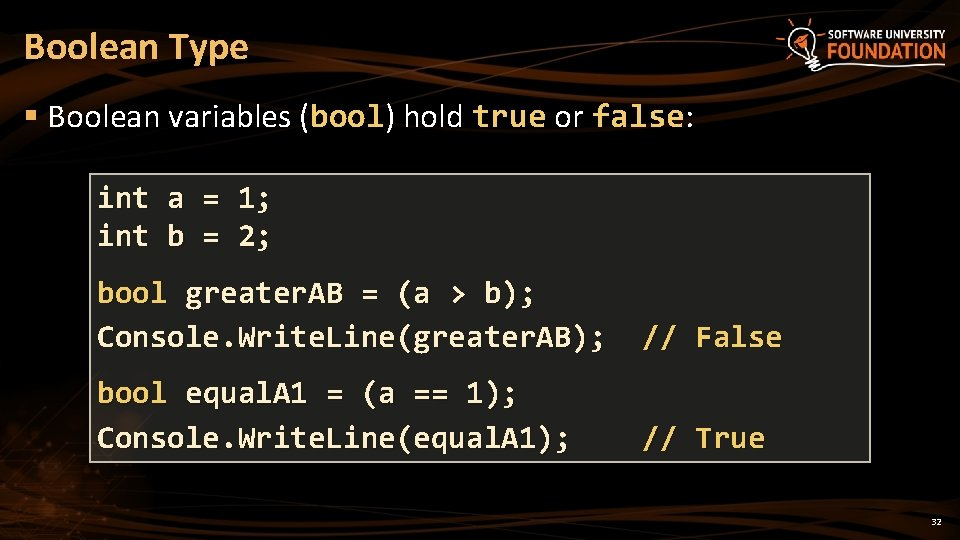Boolean Type § Boolean variables (bool) hold true or false: int a = 1; int b = 2; bool greater. AB = (a > b); Console. Write. Line(greater. AB); // False bool equal. A 1 = (a == 1); Console. Write. Line(equal. A 1); // True 32Problem: Special Numbers § A number is special when its sum of digits is 5, 7 or 11 § For all numbers 1…n print the number and if it is special 20 1 2 3 4 5 6 7 -> -> False True False True 8 -> False 9 -> False 10 -> False 11 -> False 12 -> False 13 -> False 14 -> True 15 16 17 18 19 20 -> -> -> False True False Check your solution here: https: //judge. softuni. bg/Contests/Practice/Index/171#4 33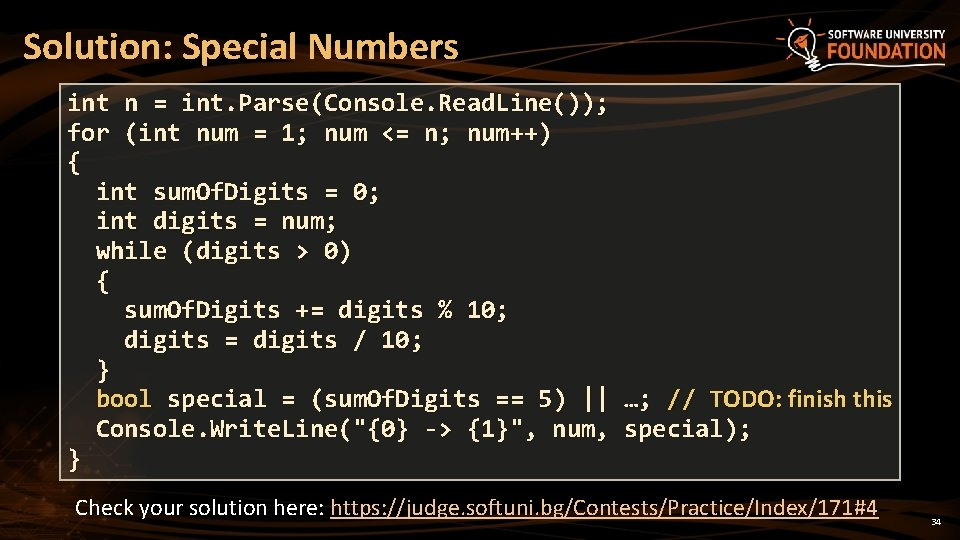Solution: Special Numbers int n = int. Parse(Console. Read. Line()); for (int num = 1; num <= n; num++) { int sum. Of. Digits = 0; int digits = num; while (digits > 0) { sum. Of. Digits += digits % 10; digits = digits / 10; } bool special = (sum. Of. Digits == 5) || …; // TODO: finish this Console. Write. Line("{0} -> {1}", num, special); } Check your solution here: https: //judge. softuni. bg/Contests/Practice/Index/171#4 34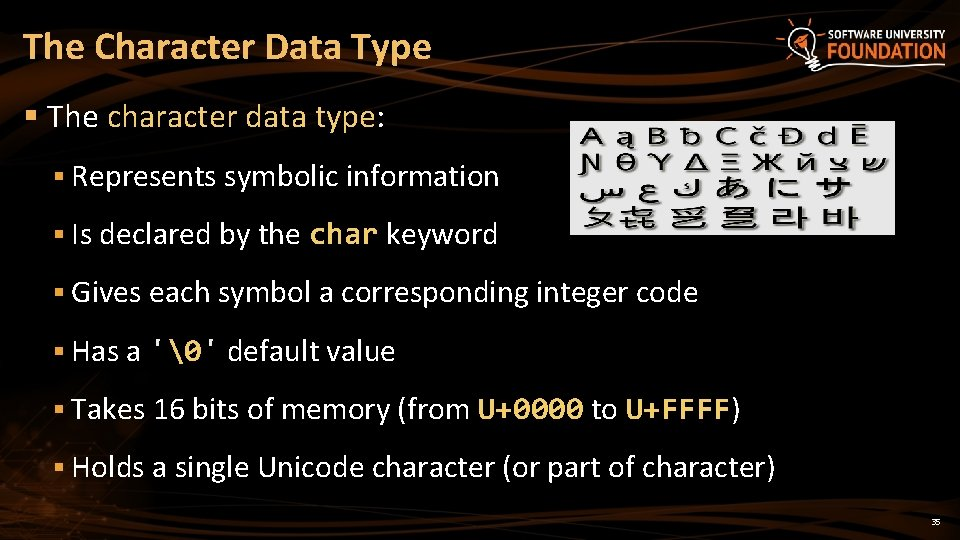The Character Data Type § The character data type: § Represents symbolic information § Is declared by the char keyword § Gives each symbol a corresponding integer code § Has a '' default value § Takes 16 bits of memory (from U+0000 to U+FFFF) § Holds a single Unicode character (or part of character) 35Characters and Codes § Each character has an unique Unicode value (int): char ch = 'a'; Console. Write. Line("The code of '{0}' is: {1}", ch, (int) ch); ch = 'b'; Console. Write. Line("The code of '{0}' is: {1}", ch, (int) ch); ch = 'A'; Console. Write. Line("The code of '{0}' is: {1}", ch, (int) ch); ch = 'щ'; // Cyrillic letter 'sht' Console. Write. Line("The code of '{0}' is: {1}", ch, (int) ch); 36Problem: Triples of Latin Letters § Write a program to read an integer n and print all triples of the first n small Latin letters, ordered alphabetically: 3 aaa aab aac aba abb abc aca acb acc baa bab bac bba bbb bbc bca bcb bcc caa cab cac cba cbb cbc cca ccb ccc Check your solution here: https: //judge. softuni. bg/Contests/Practice/Index/171#5 37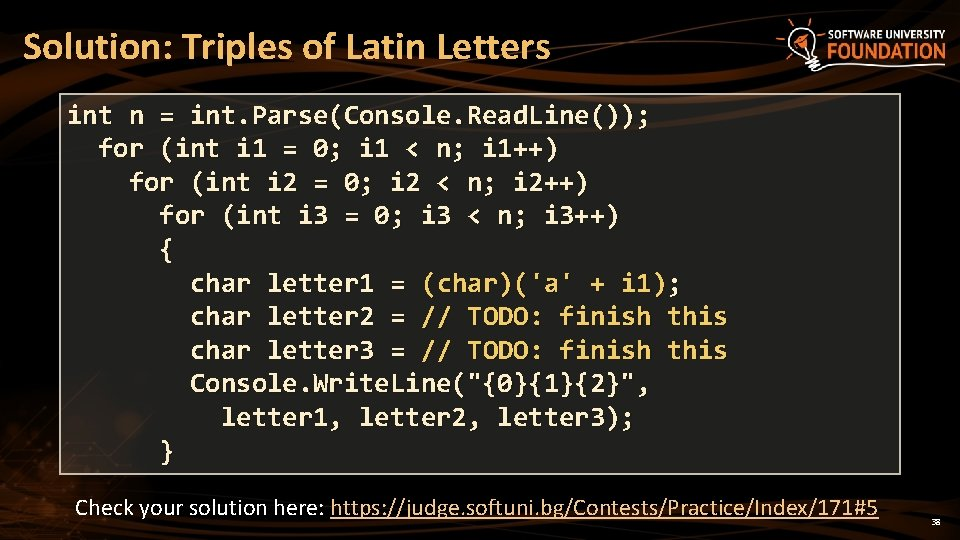Solution: Triples of Latin Letters int n = int. Parse(Console. Read. Line()); for (int i 1 = 0; i 1 < n; i 1++) for (int i 2 = 0; i 2 < n; i 2++) for (int i 3 = 0; i 3 < n; i 3++) { char letter 1 = (char)('a' + i 1); char letter 2 = // TODO: finish this char letter 3 = // TODO: finish this Console. Write. Line("{0}{1}{2}", letter 1, letter 2, letter 3); } Check your solution here: https: //judge. softuni. bg/Contests/Practice/Index/171#5 38Escaping Characters § Escaping sequences are: § Represent a special character like ', " or n (new line) § Represent system characters (like the [TAB] character t) § Commonly used escaping sequences are: § ' for single quote " for double quote § \ for backslash n for new line § u. XXXX for denoting any other Unicode symbol 39Character Literals – Example char symbol = 'a'; // An ordinary character symbol = 'u 006 F'; // Unicode character code in a // hexadecimal format (letter 'o') symbol = 'u 8449'; // 葉 (Leaf in Traditional Chinese) symbol = '''; // Assigning the single quote character symbol = '\'; // Assigning the backslash character symbol = 'n'; // Assigning new line character symbol = 't'; // Assigning TAB character symbol = "a"; // Incorrect: use single quotes! 40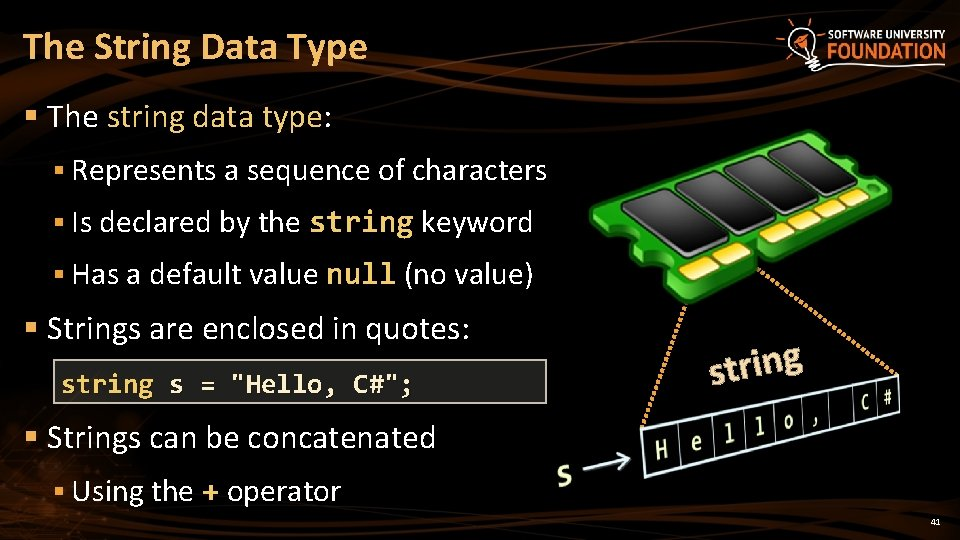The String Data Type § The string data type: § Represents a sequence of characters § Is declared by the string keyword § Has a default value null (no value) § Strings are enclosed in quotes: string s = "Hello, C#"; g n i r t s § Strings can be concatenated § Using the + operator 41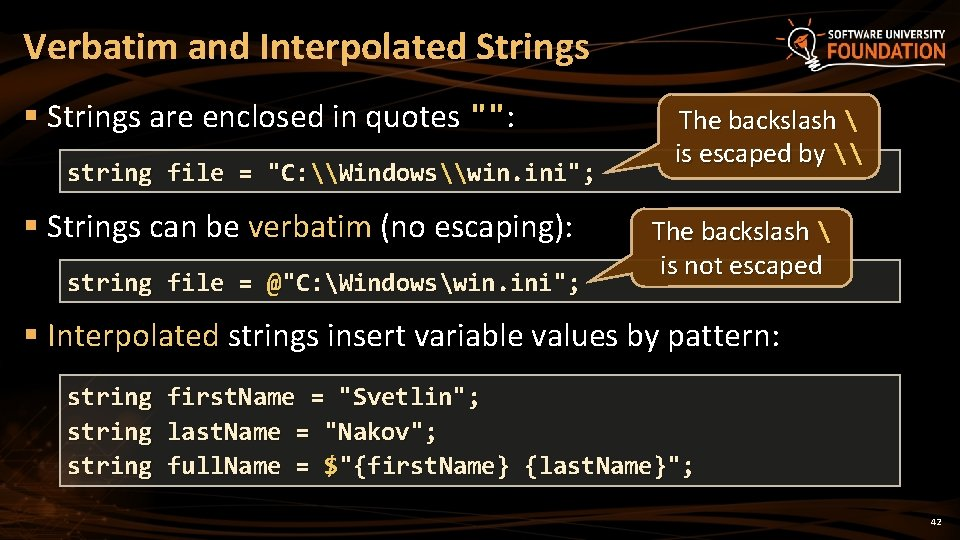Verbatim and Interpolated Strings § Strings are enclosed in quotes "": string file = "C: \Windows\win. ini"; § Strings can be verbatim (no escaping): string file = @"C: Windowswin. ini"; The backslash is escaped by \ The backslash is not escaped § Interpolated strings insert variable values by pattern: string first. Name = "Svetlin"; last. Name = "Nakov"; full. Name = \$"{first. Name} {last. Name}"; 42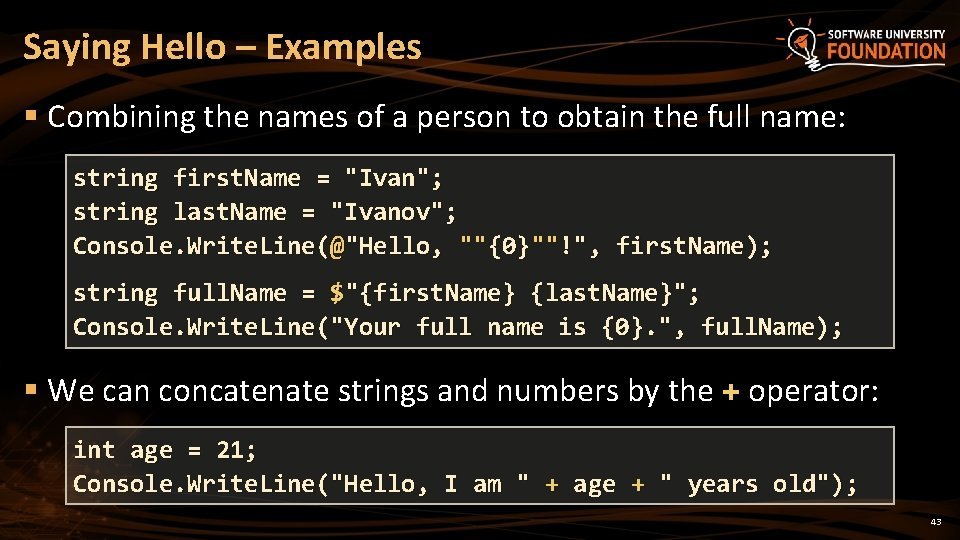Saying Hello – Examples § Combining the names of a person to obtain the full name: string first. Name = "Ivan"; string last. Name = "Ivanov"; Console. Write. Line(@"Hello, ""{0}""!", first. Name); string full. Name = \$"{first. Name} {last. Name}"; Console. Write. Line("Your full name is {0}. ", full. Name); § We can concatenate strings and numbers by the + operator: int age = 21; Console. Write. Line("Hello, I am " + age + " years old"); 43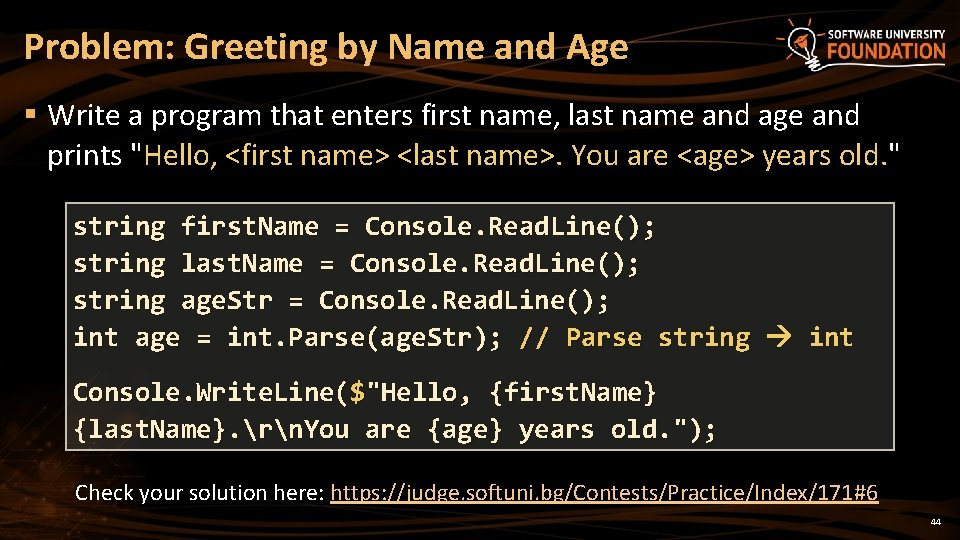Problem: Greeting by Name and Age § Write a program that enters first name, last name and age and prints "Hello, <first name> <last name>. You are <age> years old. " string first. Name = Console. Read. Line(); string last. Name = Console. Read. Line(); string age. Str = Console. Read. Line(); int age = int. Parse(age. Str); // Parse string int Console. Write. Line(\$"Hello, {first. Name} {last. Name}. rn. You are {age} years old. "); Check your solution here: https: //judge. softuni. bg/Contests/Practice/Index/171#6 44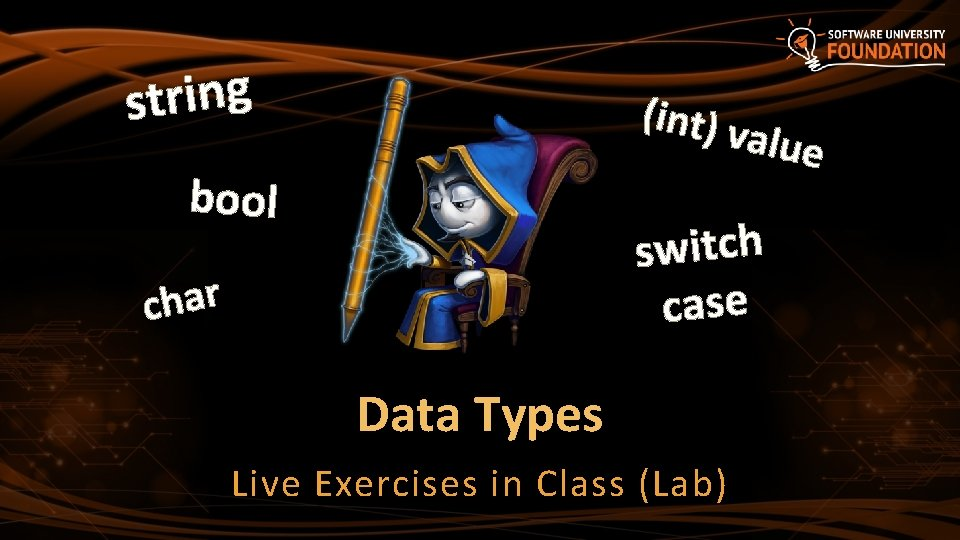g n i r t s (int) v alue bool switch case r a h c Data Types Live Exercises in Class (Lab)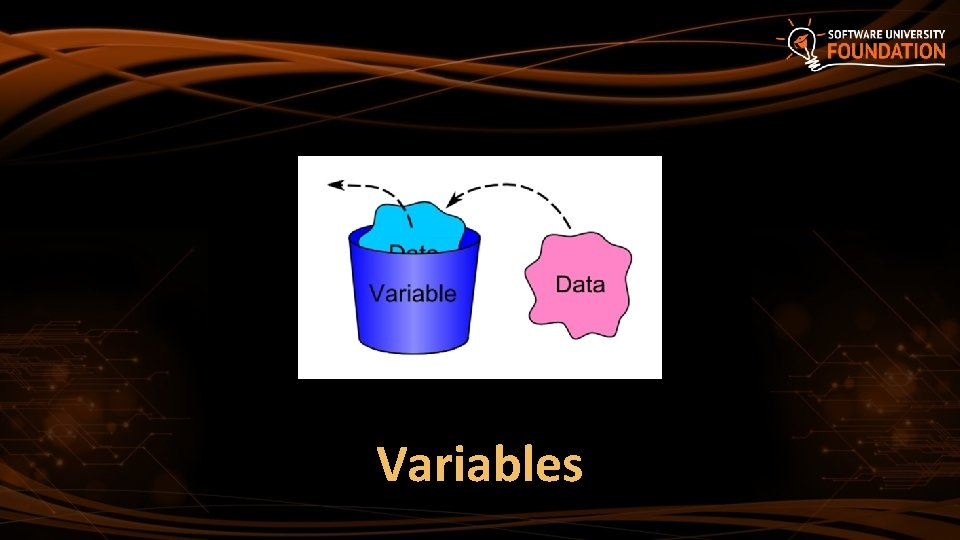VariablesNaming Variables § Variable names § Always refer to the naming conventions of a programming language – for C# use camel. Case § Preferred form: [Noun] or [Adjective] + [Noun] § Should explain the purpose of the variable (Always ask yourself "What this variable contains? ") first. Name, report, config, users. List, font. Size, max. Speed foo, bar, p, p 1, p 2, populate, Last. Name, last_name, LAST_NAME 47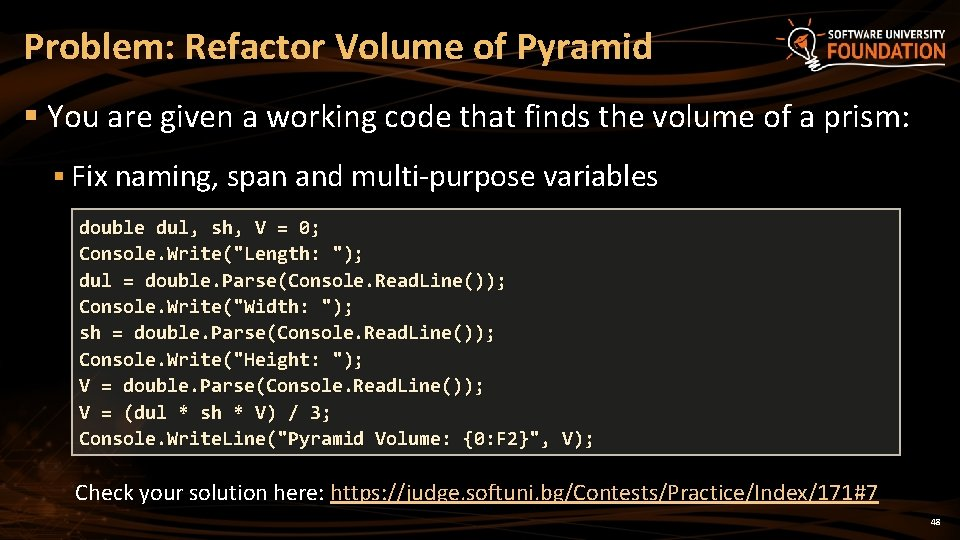Problem: Refactor Volume of Pyramid § You are given a working code that finds the volume of a prism: § Fix naming, span and multi-purpose variables double dul, sh, V = 0; Console. Write("Length: "); dul = double. Parse(Console. Read. Line()); Console. Write("Width: "); sh = double. Parse(Console. Read. Line()); Console. Write("Height: "); V = double. Parse(Console. Read. Line()); V = (dul * sh * V) / 3; Console. Write. Line("Pyramid Volume: {0: F 2}", V); Check your solution here: https: //judge. softuni. bg/Contests/Practice/Index/171#7 48Variable Scope and Lifetime § Scope shows from where you can access a variable § Lifetime shows how long a variable stays in memory static void Main() Accessible in the Main() { var outer = "I'm inside the Main()"; for (int i = 0; i < 10; i++) Accessible in the loop { var inner = "I'm inside the loop"; } Console. Write. Line(outer); // Console. Write. Line(inner); // Error } 49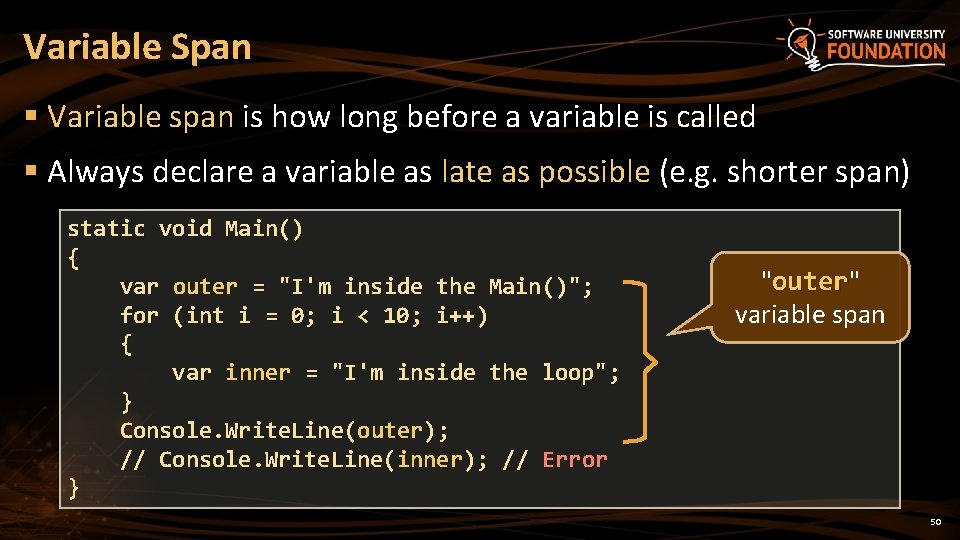Variable Span § Variable span is how long before a variable is called § Always declare a variable as late as possible (e. g. shorter span) static void Main() { var outer = "I'm inside the Main()"; for (int i = 0; i < 10; i++) { var inner = "I'm inside the loop"; } Console. Write. Line(outer); // Console. Write. Line(inner); // Error } "outer" outer variable span 50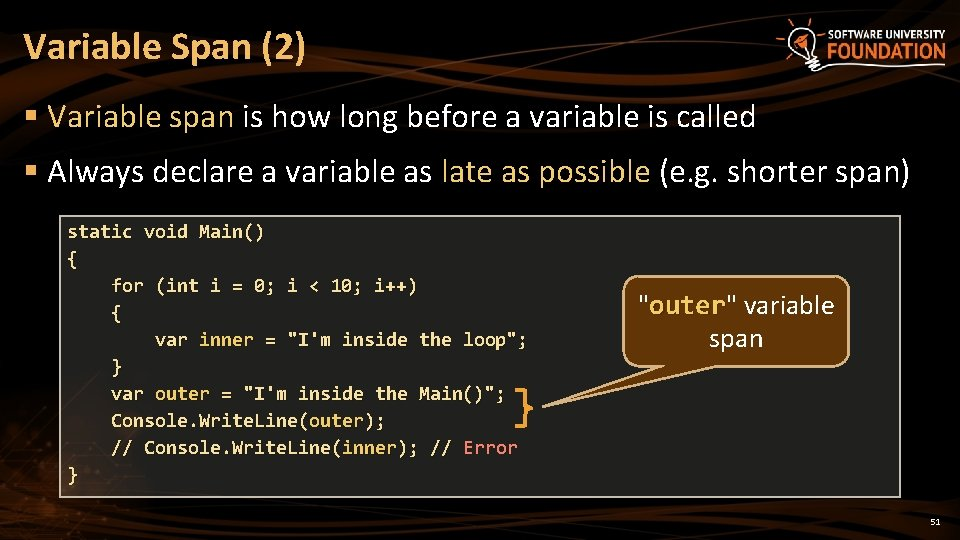Variable Span (2) § Variable span is how long before a variable is called § Always declare a variable as late as possible (e. g. shorter span) static void Main() { for (int i = 0; i < 10; i++) { var inner = "I'm inside the loop"; } var outer = "I'm inside the Main()"; Console. Write. Line(outer); // Console. Write. Line(inner); // Error } "outer" variable outer span 51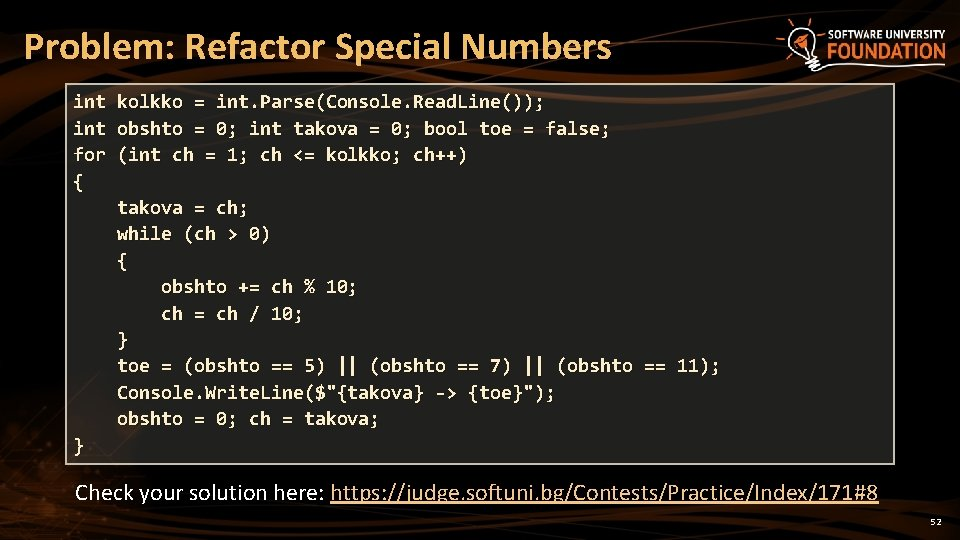Problem: Refactor Special Numbers int kolkko = int. Parse(Console. Read. Line()); int obshto = 0; int takova = 0; bool toe = false; for (int ch = 1; ch <= kolkko; ch++) { takova = ch; while (ch > 0) { obshto += ch % 10; ch = ch / 10; } toe = (obshto == 5) || (obshto == 7) || (obshto == 11); Console. Write. Line(\$"{takova} -> {toe}"); obshto = 0; ch = takova; } Check your solution here: https: //judge. softuni. bg/Contests/Practice/Index/171#8 52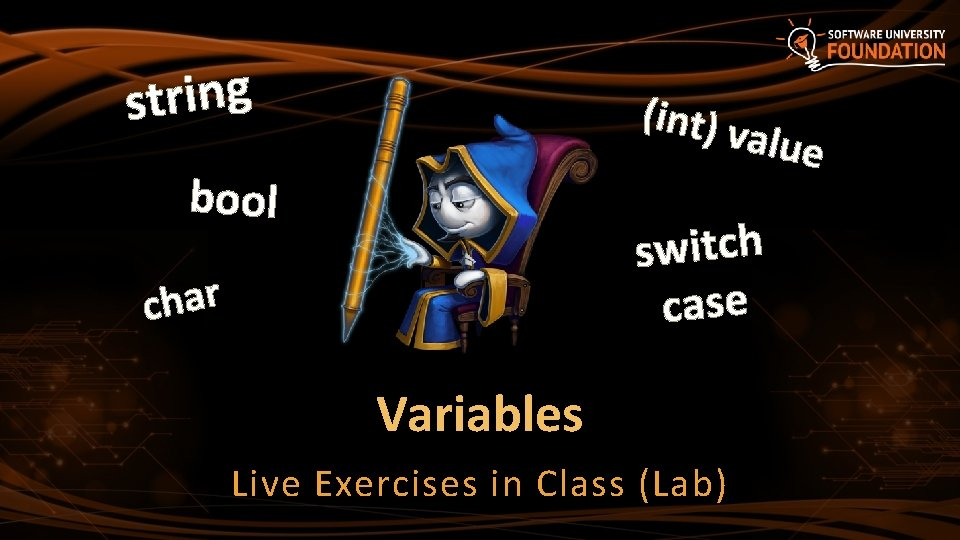g n i r t s (int) v alue bool switch case r a h c Variables Live Exercises in Class (Lab)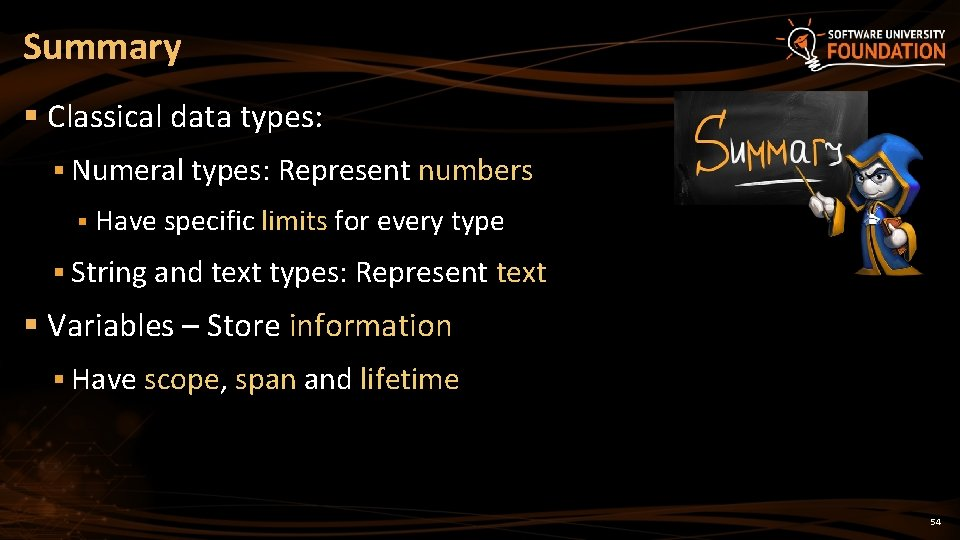Summary § Classical data types: § Numeral types: Represent numbers § Have specific limits for every type § String and text types: Represent text § Variables – Store information § Have scope, span and lifetime 54Data Types ? s n o i t s e u Q ? ? ? https: //softuni. bg/courses/programming-fundamentals/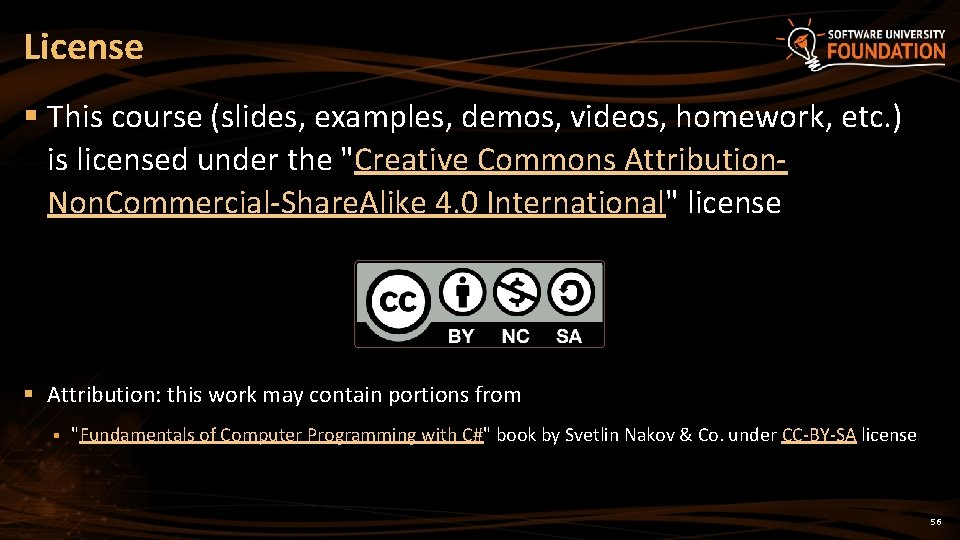License § This course (slides, examples, demos, videos, homework, etc. ) is licensed under the "Creative Commons Attribution. Non. Commercial-Share. Alike 4. 0 International" license § Attribution: this work may contain portions from § "Fundamentals of Computer Programming with C#" book by Svetlin Nakov & Co. under CC-BY-SA license 56Free Trainings @ Software University § Software University Foundation – softuni. org § Software University – High-Quality Education, Profession and Job for Software Developers § softuni. bg § Software University @ Facebook § facebook. com/Software. University § Software University @ You. Tube § youtube. com/Software. University § Software University Forums – forum. softuni. bg